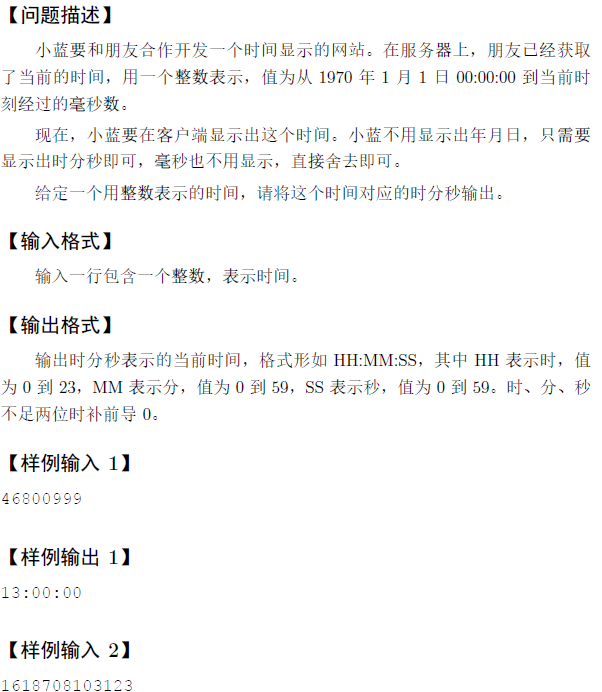# 蓝桥杯—时间显示（C语言解法）

## 题目描述## 注意：

1. 1s=1000ms
2. 输出格式，输出位数为两位，不足用0补充，格式为{"%.2d"}

## 代码

``````#include<stdio.h>
int main(){
int tem,day=0,hour=0,min=0,sec=0;
long long time;
scanf("%lld",&time);
day=time/(24*60*60*1000);//求出一共有多少整天
tem=time%(24*60*60*1000);//求出有多少不足一天的毫秒数
hour=tem/(1000*60*60);
min=(tem%(1000*60*60))/(1000*60);
sec=(tem%(1000*60))/(1000);
printf("%.2d:%.2d:%.2d",hour,min,sec);
return 0;
}
``````

### 推荐这些文章：

2<=N<=100000,0<=Ai<=10^9

5
2 6 4 10 20

10

#include<stdio.h>
int nm;
int main(){
int i,sum=1,he=0;
nm=1;
for(i=2;i<=20;i++){
sum*=2;
nm[i]=sum;
}
for(i=1;i<=20;i++){
he+=nm/n...

#include<bits/stdc++.h>
using namespace std;
int main()
{
long long day = 24*60*60*1000;
long long time;
ios::sync_with_stdio(false);
cin >> time;
time...

#include<stdio.h>
int runyear(int year){//判断是否为闰年
if(year%4==0 && year%100!=0 ||year%400==0)return 1;
else return 0;
}
int main(){
int day={0,31,28,31,30,31,30,31,31,30,31,30,31};
int tian=1,year=20...

#include<stdio.h>
long long int res;//!!注意类型，int型会错，ll型才行
int cnt=0;
int count;
int main(){
long long int n...

1 1
1 2 3

4

C语言显示当前时间

#include<stdio.h>
#include<Windows.h>
#include<time.h>

struct clock {
int hour;
int minute;
int second;
};

typedef struct clock CLOCK;

int main()
{
CLOCK t = { 0,0,0 };
int n = 100, i = 0;
char c;
time_t now;
struct tm local;
while (1)
{
time(&now);
localtime...

C语言中没有保留到小数点多少位的函数或者方法，所以要换一种思路

#include<stdio.h>
int main(){
int i;
long long res,a=1,b=3,tem;
for(i=1;i<2000;i++){
res=(10000000*a)/b;
if(res%10>4){
res=res/10+1;
}
else res=r...

THE END